#### Power in AC Circuits II, Instantaneous and Average Power

Power Dissipation in an AC circuit:

In common, an ac circuit will have a combination of resistive and reactive components and the reactive elements might be either capacitive or inductive as shown in figure below. This signifies that at different points in the circuit the voltage and current relationships will differ depending on the elements included. From the point of view of a voltage source driving such a circuit, the overall network will have impedance, that has a phase and magnitude and a current will flow into the circuit which as well possesses a corresponding phase and magnitude as shown below.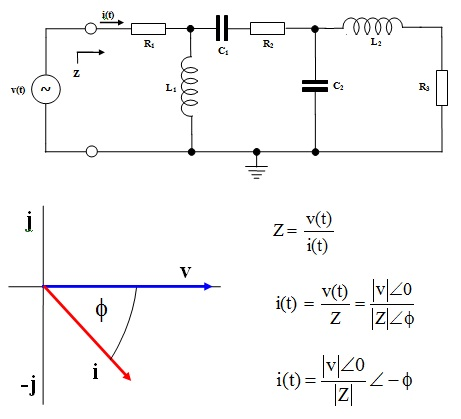Figure: The Phase Relationship Associated with an AC Circuit having Reactance

A plot of voltage that is taken as the reference zero angle, and the current with instantaneous power is shown in figure below. The current is observed to lag behind the voltage by an angle . Note that, dissimilar the case for purely and resistive reactive circuits, the instantaneous power profile is not symmetrical. This can be seen in this illustration that the power profile is positive for longer than it is negative and as well that it reaches a higher positive peak than negative peak. This signifies that more power is delivered to the network in each and every cycle of the sinusoidal source than is returned to source. Thus there is a net transfer of power from the source to circuit and this power is dissipated in the resistive components of network.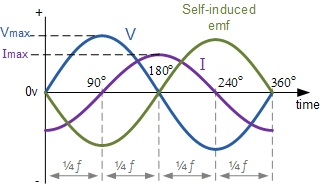Figure: Waveforms showing Power Relations in an AC Circuit containing Reactance

Instantaneous Power:

The instantaneous power can be found as before as the product of current and voltage as continuous functions of time:

If, v(t) = Vm sin ωt and i(t) = Im sin (ωt - Φ)

Then,

Pi = Vm Im sin ωt. sin (ωt - Φ)

Average Power:

PAVE = (1/T) 0T Pidt = (1/T) 0Tv(t) i(t) dt

PAVE = (1/T) 0T VmIm Sin ωt . Sin (ωt - Φ) dt

PAVE = (VmIm/T) 0∫T Sin ωt . Sin (ωt - Φ) dt

By using the trigonometric expansion:

sinA sinB = ½[cos(A - B) – cos(A + B)] where, A = ωt and B = ωt – Φ

PAVE = (VmIm/2T) 0∫T [Cos Φ - Cos (2ωt - Φ)] dt

By using the trigonometric expansion:

Cos (A + B) = cosA cosB - sinA sinB where, A = 2ωt and B = – Φ

It gives:

PAVE = (VmIm/2T) 0T [Cos(Φ) - Cos(2ωt) Cos(-Φ)+ Sin(2ωt) Sin(-Φ)] dt

But, cos(-Φ) = cos Φ and  sin(-Φ) = -sin Φ

And hence,

PAVE = (VmIm/2T) 0T [CosΦ - Cos 2ωt . Cos Φ - Sin 2ωt Sin Φ] dt

The factors cos Φ and sin Φ is constants for a given circuit where there is a given phase shift between the supply current and voltage drawn by the circuit and hence: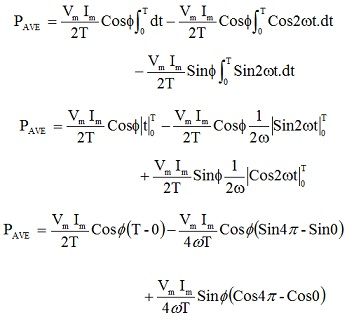The last two terms in this expression encompass a value of zero as prior to and hence finally:

PAVE = (Vm Im/2) cos Φ = (Vm/√2) (Im/√2) cos Φ

PAVE = VRMS IRMS cos Φ

The term Cos Φ is termed to as Power Factor of the circuit. This is a property of ac network and is recognized by the phase angle of network impedance.

Power Factor = cos Φ

Power Factor varies between the value of 0 and 1.

Φ = 0o => cos Φ = 1 PAVE = VRMS IRMS (Purely resistive circuit)

Φ = 90o => cos Φ = 0 PAVE = 0 (Purely reactive circuit)

The average power computed above is the real power consumed from the power delivered to network. It is dissipated by the resistive elements of circuit. Though, the source should be rated to produce and deliver the total power demanded by the circuit even although not all of this is consumed. The power dissipated is as well termed to as Active Power and symbolizes energy consumed.

Complex Power:

This has been seen from the prior waveform exhibiting the instantaneous power that the positive excursion is bigger than the negative excursion, and hence there is a net transfer of power from the source to load per cycle of the source voltage. Phase of the impedance of network outcomes in a phase angle between current and voltage which gives the Power Factor in the Average or Active Power drawn by the network. Though, as with purely reactive circuits, there is as well some power that is drawn from the source, stored temporarily in the reactive elements and then returned to the source in the later part of each and every cycle. This is termed to as Reactive Power. In practice, the source driving the network should be rated to handle and deliver both active and reactive power, even although only the active power will be dissipated by circuit. The vector sum of Active and Reactive Power is termed to as the Apparent Power and offers the concept of Complex Power as shown in phasor form in figure below.

Apparent Power = Active Power + j Reactive Power

Apparent Power = Average Power + j Reactive Power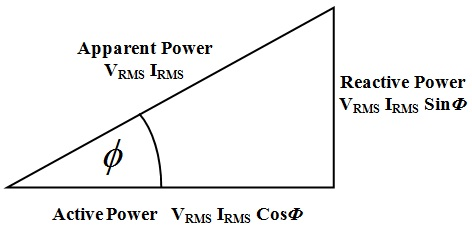Figure: A Phasor representation of Complex Power

Apparent Power = VRMS IRMS = (Vm Im)/2

Active Power or Average Power = VRMS IRMS cos Φ

Reactive Power = j VRMS IRMS sin Φ

V2RMS I2RMS = V2RMS I2RMS cos2 Φ + V2RMS I2RMS sin2 Φ

In order to avoid having to encompass a source that should be capable of providing much more power than is really going to be consumed by a network, the goal is to reduce the amount of reactive power demanded of source. Thus the aim is to make the apparent power and the active power equivalent. This signifies making the power factor as close to unity as possible.

Let consider the network impedance as shown in figure below:

Z = R + jX

Sin Φ = X/|Z|

Cos Φ = R/|Z|

Power factor = Cos Φ = R/|Z|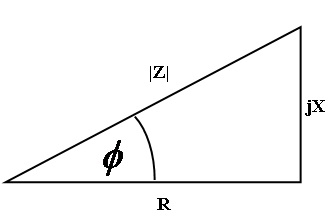Figure: Power Factor in Complex Power

Where R is the total equivalent resistance of ac network as seen by the source. This might not really be a resistive element however represent can work done by some piece of machine or equipment that is provided with electrical power and consumes energy.

Z = 193 + j 236 - j 32 Ω

↑          ↑             ↑

Resistance  Inductive    Capacitive Reactance

Reactance

Current flowing into the circuit from the source can be determined as:

i = V/Z = (|V|∠0o)/(|Z|∠ΦZ) = 220∠0o/281∠46.6o = 0.78 ∠46.6o A

Power factor of the network is shown as:

Power factor = cos Φ = cos 46.6o = 0.687

Complex power can be computed as:

Apparent Power = VRMS IRMS = (Vm Im)/2 = (220 x 0.78)/2 = 85.8 W

Active power = VRMS IRMS = [(Vm Im)/2] cos Φ = 85.8 x 0.687 = 58.9 W

Reactive Power = j VRMS IRMS sin Φ = j [(Vm Im)/2] sin Φ = j 85.8 x 0.727 = j 62.4 W

Latest technology based Electrical Engineering Online Tutoring Assistance

Tutors, at the www.tutorsglobe.com, take pledge to provide full satisfaction and assurance in Electrical Engineering help via online tutoring. Students are getting 100% satisfaction by online tutors across the globe. Here you can get homework help for Electrical Engineering, project ideas and tutorials. We provide email based Electrical Engineering help. You can join us to ask queries 24x7 with live, experienced and qualified online tutors specialized in Electrical Engineering. Through Online Tutoring, you would be able to complete your homework or assignments at your home. Tutors at the TutorsGlobe are committed to provide the best quality online tutoring assistance for Electrical Engineering Homework help and assignment help services. They use their experience, as they have solved thousands of the Electrical Engineering assignments, which may help you to solve your complex issues of Electrical Engineering. TutorsGlobe assure for the best quality compliance to your homework. Compromise with quality is not in our dictionary. If we feel that we are not able to provide the homework help as per the deadline or given instruction by the student, we refund the money of the student without any delay.